# How do I get started using Mathematica from Excel?

Mathematica Link for Excel enables you to share data and calculations between Mathematica and Microsoft Excel.

Here we showcase the first steps in connecting to Mathematica from Excel. For connecting to Excel from Mathematica, see here.

Advanced examples and information can be found in the Mathematica Link for Excel documentation.

### Installing the Add-In in Excel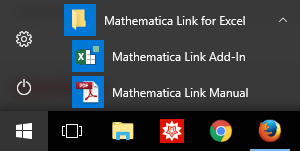3. Verify that the Mathematica Link for Excel toolbar is present.
4.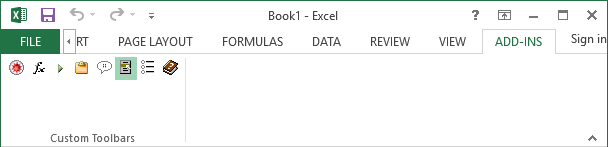Mathematica Link for Excel is now installed in Excel.

### Running a Mathematica Function in Excel

Enter calls to Mathematica in the cell content slot using the format:

``=EVAL("FunctionName", arg1, arg2, ...)``

In the following example, we compute the determinant of the matrix defined in cells A1 through B2.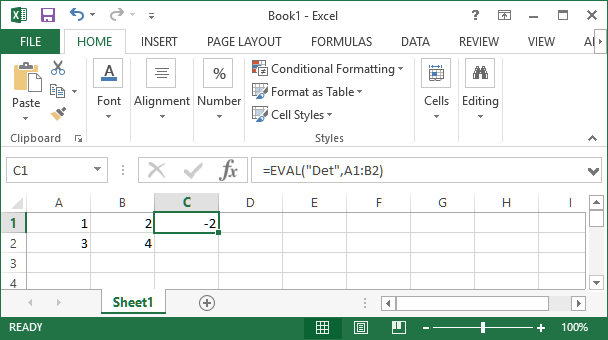### Using the Function Wizard for Building Function Calls

The Mathematica Link for Excel toolbar contains a Function Wizard to help with more complicated Wolfram Language function calls in Excel.

To use the Function Wizard:

1. Open the wizard by clicking the “fx” icon on the toolbar.
2.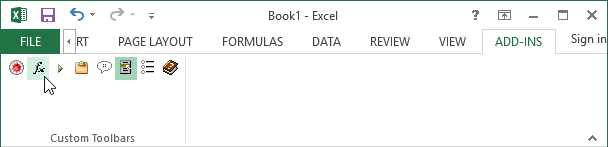3. Find the Wolfram Language function you wish to use. Click Next to confirm.
4.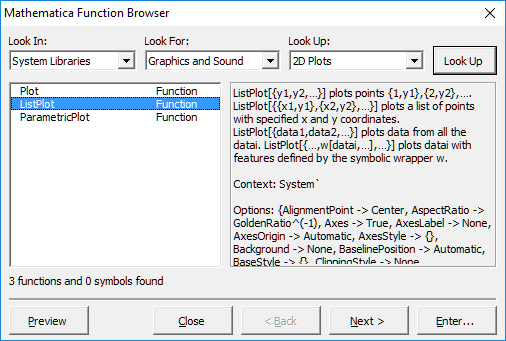5. Enter the arguments for the function. Click Next to confirm.
6.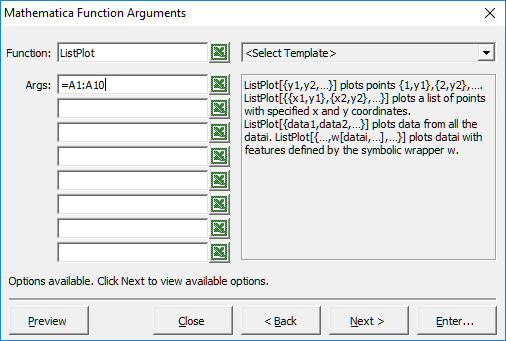7. If needed, alter any of the default options for the function. Click Enter to use the function.
8.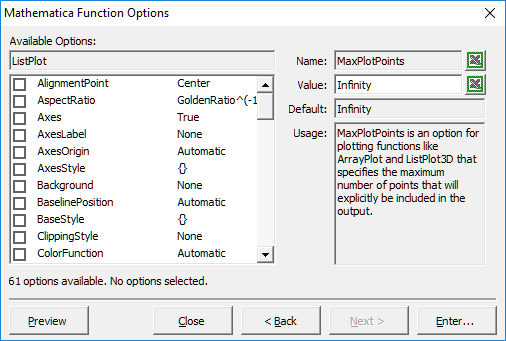9. Enter the ID of the cell you want the output to appear in.
10.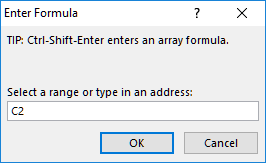The output will then be placed in the specified cell: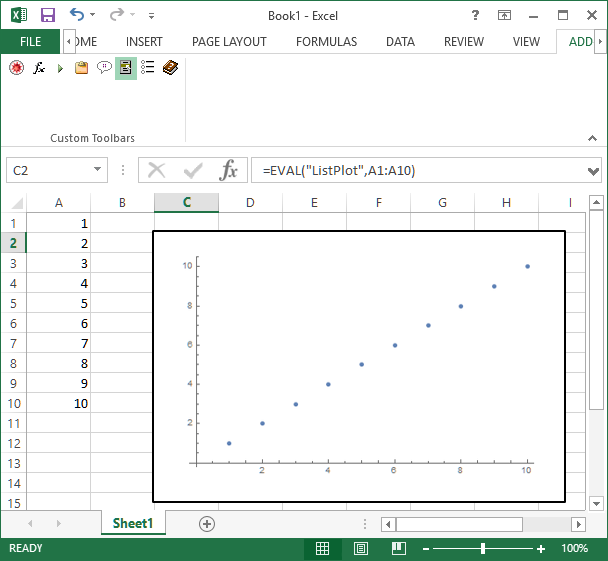설명이 도움이 되었나요?

하실 말씀이 있습니까?

피드백 감사합니다.

## 지원 문의

청구서, 제품 동기화에 관한 질문에서 기술적인 질문까지 부담없이 문의하세요.전화하기

월요일 - 금요일
8am–5pm 중부 표준시

• 제품 등록 및 동기화
• 구매 전 정보 및 주문
• 설치 및 동작

## 고급 기술지원 (해당 고객을 대상으로)

월요일 - 목요일
8am–7pm 중부 표준시

금요일
8:30–10am & 11am–5pm 중부 표준시

• 우선적 기술지원
• Wolfram 전문가들의 제품 지원
• Wolfram 프로그래밍
• 고급 설치 지원